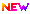High Impact Factor : 4.396| Submit Manuscript Online|

## Master yr maths

### Author(s):

Patel Anuj P. , Alpha college of engg.& tech.; Ajaykimar T. Shah, Alpha college of engg.& tech.

Master YR Maths

### Abstract

A master yr maths is a game whose rules, strategies, and outcomes are defined by clear mathematical parameters. Often, such games have simple rules and match procedures, such as Tic-tac-toe and Dots and Boxes. Generally, mathematical games need not be conceptually intricate to involve deeper computational underpinnings. For example, even though the rules of Mancala are relatively basic, the game can be rigorously analyzed through the lens of combinatorial game theory. Mathematical games differ sharply from mathematical puzzles in that mathematical puzzles require specific mathematical expertise to complete, whereas mathematical games do not require a deep knowledge of mathematics to play. Often, the arithmetic core of mathematical games is not readily apparent to players untrained to note the statistical or mathematical aspects some mathematical games are of deep interest in the field of recreational mathematics when studying a game's core mathematics, arithmetic theory is generally of higher utility than actively playing or observing the game itself. To analyze a game numerically, it is particularly useful to study the rules of the game insofar as they can yield equations or relevant formulas. This is frequently done to determine winning strategies or to distinguish if the game has a solution.

### Other Details

Paper ID: IJSRDV6I110259
Published in: Volume : 6, Issue : 11
Publication Date: 01/11/2019
Page(s): 447-448